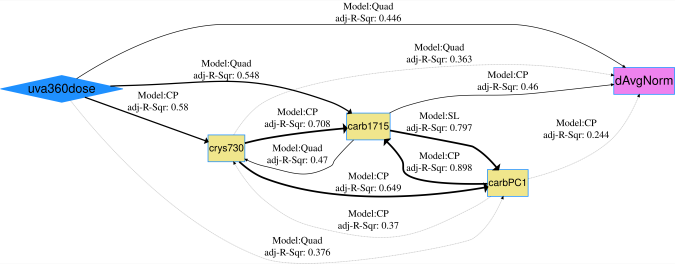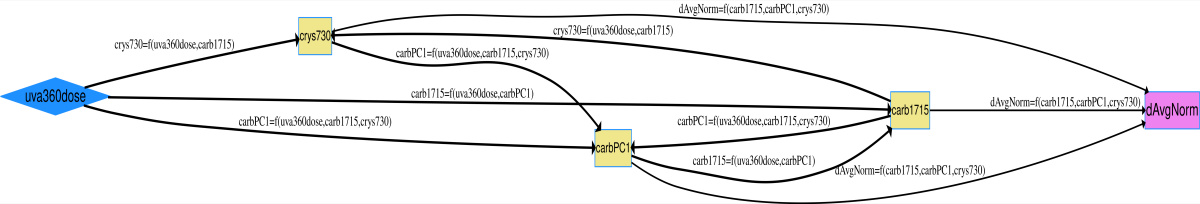# Quantifying and Modeling Photovoltaic Backsheet Cracking

## Data Description

A dataset of cracks in photovoltaic backsheets with inner layers of either ethylene-vinyl acetate or polyethylene exposed to 4,000 hours of continuous UVA irradiance with an intensity of 1.55 $$w/m^2$$ at 340 $$nm$$ and a chamber temperature of 70$$^\circ$$C. See the associated journal article in Polymer Degradation and Stability for details on obtaining quantitative crack measurements [@klinke_2018_non-destructive].

97 observations of 5 variables are included:

• Response - dAvgNorm: average crack depth normalized by the backsheet’s inner layer thickness, dimensionless.
• Stress - uva360dose: cumulative, integrated irradiance dose received by the sample for all wavelengths $$<$$ 360 $$nm$$, in $$MJ/m^2$$.
• Mechanism - crys730: percent crystallinity of polyethylene regions determined from the ratio of Fourier transform infrared (FTIR) spectroscopy peak intensities at 731 and 720 $$cm^{-1}$$ [@huang_attenuated_1992], dimensionless.
• Mechanism - carb1715: ketone carbonyl index determined from the ratio of FTIR peak intensities at 1715 and 2851 $$cm^{-1}$$, dimensionless.
• Mechanism - carbPC1: 1st principal component score for the carbonyl region between 1500 and 1800 $$cm^{-1}$$.

## Load data and run code to build netSEM

## Load the crack data set and preview column metadata
data(crack)
?crack

## Run netSEMp1 model
ans1 <- netSEMp1(crack)
## Plot the network model for principle 1
plot(ans1, cutoff = c(0.4, 0.5, 0.6))

## Run netSEMp2 model
ans2 <- netSEMp2(crack)
## Plot the network model for principle 2
plot(ans2, cutoff = c(0.4, 0.5, 0.6))

## Network diagram for data

dAvgNorm is the endogenous and all other variables are considered as exogenous. Based on the plot, the strongest pathway appears to be uva360dose $$\rightarrow$$ carb1715 $$\rightarrow$$ dAvgNorm. This can be confirmed using the pathwayRMSE() function.Crack netSEMp1 modelCrack netSEMp2 model

## Reference

Huang, J. B., J. W. Hong, and M. W. Urban. “Attenuated Total Reflectance Fourier Transform Infra-Red Studies of Crystalline-Amorphous Content on Polyethylene Surfaces.” Polymer (1992) 33: 5173–78. https://doi.org/10.1016/0032-3861(92)90797-Z.

Klinke, Addison G, Abdulkerim Gok, Silas I Ifeanyi, and Laura S Bruckman. “A Non-Destructive Method for Crack Quantification in Photovoltaic Backsheets Under Accelerated and Real-World Exposures.” Polymer Degradation and Stability (2018) 153: 224–54. https://doi.org/10.1016/j.polymdegradstab.2018.05.008.